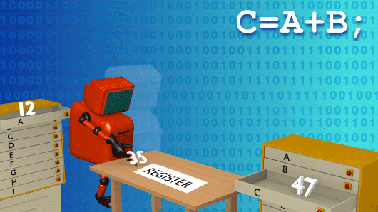Programming Basics

Learn basic computer programming skills and master the art of writing C/C++ programs to solve real world problems.This course is archived
Estimated 9 weeks
6–8 hours per week
Self-paced
Free

Basic concepts of computer programming are introduced, starting with the notion of an algorithm. Emphasis is on developing the ability to write programs to solve practical computational problems.

Topics include:

• Algorithms
• Elements of C/C++ programming languages
• Basic data types
• Sequential and conditional execution
• Iterative solutions
• Arrays, matrices and their applications
• Functions
• Sorting and searching
• Elements of string processing
• Introduction to pointers
• Basics of Software Engineering
• Structures
• File Processing

Learners will read and understand many sample programs, and will have to write several on their own. This course deals with basic programming, and sets the foundation for solid programming practices for beginners.

This course is part of the Fundamentals of Computer Science XSeries Program:

At a glance

• Institution: IITBombayX
• Subject: Computer Science
• Level: Introductory
• Prerequisites:

Knowledge of high school mathematics is essential and adequate. Exposure to pre-calculus is desirable.

What you'll learn

Skip What you'll learn
• Basic programming skills
• To write C/C++ programs to solve real world computational problems.
• Good programming practices
• How to handle large and complex programs.